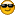###Author Topic: Problem on exit from NASM dll with callback, called from Python ctypes  (Read 3953 times)

####paml27

• Jr. Member
•• Posts: 36##### Problem on exit from NASM dll with callback, called from Python ctypes
« on: April 19, 2018, 04:21:54 PM »
This question involves Python ctypes, but the DLL is written in NASM-64 and I thought someone on this forum would know what the answer is.  It may be strictly ctypes, or it may be related to the NASM code.

I have a simple test dll written in NASM to be called from Python ctypes, and it has a callback to Python from NASM. The callback function works correctly through a small sample set of 10 data points (I know that by stepping through the code in Visual Studio line-by-line).  On exit from the dll (after 10 iterations), VS crashes with exception code c0000005, which is an access violation.

But when I remove the call to the callback function pointer from the dll code, the program exits normally. So the problem of crashing on exit is seems to be related to the callback.

Here's the Python ctypes code:

def SimpleTestFunction_asm(X):

Input_Length_Array = []
Input_Length_Array.append(len(X)*CA_X = (ctypes.c_double * len(X))(*X)

length_array_out = (ctypes.c_double * len(Input_Length_Array))(*Input_Length_Array)

hDLL = ctypes.WinDLL("C:/Test_Projects/SimpleTestFunction/SimpleTestFunction.dll")
CallName = hDLL.Main_Entry_fn
CallName.argtypes = [ctypes.POINTER(ctypes.c_double),ctypes.POINTER(ctypes.c_double),ctypes.POINTER(ctypes.c_longlong)]
CallName.restype = ctypes.POINTER(ctypes.c_int64)

Free_Mem = hDLL.FreeMem_fn
Free_Mem.argtypes = [ctypes.POINTER(ctypes.c_double)]
Free_Mem.restype = ctypes.c_int64
start_time = timeit.default_timer()

#__________
#The callback function

LibraryCB = ctypes.WINFUNCTYPE(ctypes.c_double, ctypes.c_int64)

def LibraryCall(a):
b = math.ceil(a)
return (b)

lib_call = LibraryCB(LibraryCall)
lib_call = ctypes.cast(lib_call,ctypes.POINTER(ctypes.c_longlong))

#__________

ret_ptr = CallName(CA_X, length_array_out, lib_call)

a = ret_ptr[:2]
n0 = ctypes.cast(a,ctypes.POINTER(ctypes.c_int64))
n0_size = int(a[0+1] /x0 = n0[:n0_size]
free_confirm0 = Free_Mem(n0) #**** free the memory

Here's the dll code in NASM:

[BITS 64]

[default rel]
export Main_Entry_fn
export FreeMem_fn

extern malloc, realloc, free

section .data align=16
a: dq 0.0
b: dq 0
data_master_ptr: dq 0
X_ptr: dq 0
X_length: dq 0
X: dq 0
collect_ptr: dq 0
collect_length: dq 0
initial_dynamic_length: dq 0
collect: dq 0
x_var: dq 0.0
loop_counter_401: dq 0
const_40: dq 4.0
collect_ctr: dq 0
X_ctr: dq 0
Return_Pointer_Array: dq 0, 0
Input_Length_Array: dq 0, 0,
CB_Pointer: dq 0 ; the callback pointer

section .text

SimpleTestFunction_fn:
xor rcx,rcx
mov [loop_counter_401],rcx
label_401:
lea rdi,[rel X_ptr]
mov rbp,qword [rdi] ; Pointer
mov rcx,[loop_counter_401]
mov rax,80 ;[X_length]
cmp rcx,rax
jge exit_label_for_SimpleTestFunction_fn
movsd xmm0,qword[rbp+rcx]
movsd [x_var],xmm0

movsd xmm1,[const_40]
mulsd xmm0,xmm1
movsd [a],xmm0

movsd xmm0,[a]
call [CB_Pointer] ; The call to the callback function
mov [c],rax

push rbp
lea rdi,[rel collect_ptr] ; Pointer
mov rbp,qword [rdi]
mov rcx,[collect_ctr]
mov rax,qword [c]
mov [rbp+rcx],rax
pop rbp

mov [collect_ctr],rcx
mov rax,[loop_counter_401]
mov [loop_counter_401],rax
jmp label_401
; __________
label_900:
exit_label_for_SimpleTestFunction_fn:
mov rdi,Return_Pointer_Array
mov rax,qword[collect_ptr]
mov [rdi+0],rax
mov rax,qword[collect_ctr]
mov [rdi+8],rax
mov rax,rdi
ret
;__________
;Free the memory

FreeMem_fn:
sub rsp,40
call free
ret
; __________
; Main Entry

Main_Entry_fn:
push rdi
push rbp
mov [X_ptr],rcx
mov [data_master_ptr],rdx
mov [CB_Pointer],r8
; Now assign lengths
lea rdi,[data_master_ptr]
mov rbp,[rdi]
xor rcx,rcx
movsd xmm0,qword[rbp+rcx]
cvttsd2si rax,xmm0
mov [X_length],rax
; __________
; malloc for dynamic arrays
lea rdi,[data_master_ptr]
mov rbp,[rdi]
movsd xmm0,qword[rbp]
cvttsd2si rax,xmm0
mov [initial_dynamic_length],rax
mov rcx,qword[initial_dynamic_length] ; Initial size
xor rax,rax
sub rsp,40
call malloc
mov qword [collect_ptr],rax
mov rax,[initial_dynamic_length]
mov [collect_length],rax
; __________
call SimpleTestFunction_fn
exit_label_for_Main_Entry_fn:
pop rbp
pop rdi
ret

NOTE:  the two areas with the smiley-face icon are the number 8.  I don't know why it shows that way here.

Thanks very much for any ideas on how to get this to exit properly.

« Last Edit: April 19, 2018, 05:07:38 PM by paml27 »

####paml27

• Jr. Member
•• Posts: 36##### Re: Problem on exit from NASM dll with callback, called from Python ctypes
« Reply #1 on: April 20, 2018, 07:05:33 PM »